# Common Forces in Mechanics

• Friction

## Common forces in Mechanics

Contact & Non-contact forces
External force comes into picture when the body starts moving or comes to rest either by coming in contact with the body or without being in contact.

Contact force- Force applied by coming in contact with the body
Example: hitting of cricket ball with bat in game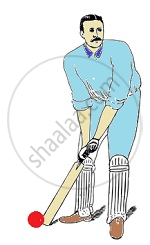Non-contact force- Force applied without coming in contact with body
Example: Coin attracted towards magnet (magnetic force)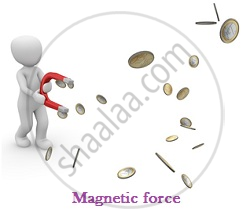Ball dropped at height attracted towards the earth (gravitational force)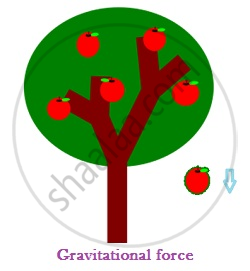Weight of a body: It is the force with which earth attracts a body towards its centre. If M is a mass of body and g is acceleration due to gravity, weight of the body is mg in vertically downward direction.

Normal Force: If two bodies are in contact a contact force arises, if the surface is smooth the direction of force is normal to the plane of contact. We call this force as Normal Force.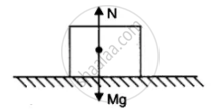Example: Let us consider a book resting on the table. It is acted upon its weight in vertically downward direction and is at rest. It means there is another force acting on the block in opposite direction, which balances its weight. This force is provided by the table and we call it as normal force.

Tension in string: Suppose a block is hanging from a string. Weight of the block is acting vertically downward but it is not moving, hence its weight is balanced by a force due to string. This force is called ‘Tension in string’. Tension is a force in a stretched string. Its direction is taken along the string and away from the body under consideration.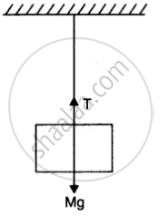Simple pulley
Consider two bodies of masses m1 and m2 tied at the ends of an extensible string, which passes over a light and friction less pulley. Let m1 > m2. The heavier body will move downwards and the lighter will move upwards. Let a be the common acceleration of the system of two bodies, which is given by
a=((m_1 - m_2)g)/(m_1 + m_2)
Tension in the string is given by
T=(2m_1m_2 × g)/(m_1+m_2)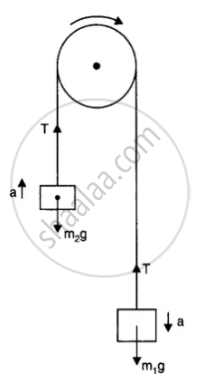Apparent Weight and Actual Weight:
Apparent weight of a body is equal to its ‘actual weight’ if the body is either in a state of rest or in a state of uniform motion.

"Apparent weight of a body for vertical upward accelerated motion is given as
Apparent weight= Actual weight ma = m(g+a)"

"Apparent weight of a body for vertically downward accelerated motion is given as
Apparent weight= Actual weight ma = m(g-a)"

### Friction

• Friction is a contact force that opposes relative motion.

• No friction exists till an external force is applied.

Angle of Friction:-
The angle made by the resultant reaction force with the vertical (normal reaction) is known as the angle of the friction.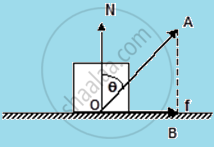Now, in the triangle OAB

(AB)/(OB) = cot  θ

So, OB = (AB)/ (cot  θ) = AB tan  θ

Or, tan θ = (OB)/(AB) = f / N

So, tan θ=f / N = µs

Angle of Repose:-
"It is the angle which an inclined plane makes with the horizontal so that a body placed over it just begins to slide of its own accord."

Consider a body of mass m resting on an inclined plane of inclination q. The forces acting on the body are shown –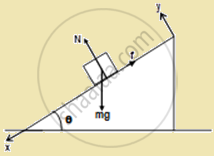F_f being the force of friction. If friction is large enough, the body will not slide down.
Along x: mg sin θ – f = 0 …(1)
Along y: N –mg cos θ = 0 …(2)
i.e. N = mg cos θ and f = mg sin θ

Thus, f ≤ µs N gives,
mg sin θ ≤ mg cos θ

So, tan θ ≤ µs . This signifies, the coefficient of static friction between the two surfaces, in order that the body doesn’t slide down.
When q is increased, then tan θ >. Thus sliding begins, and the angle θr = tan-1 µ. This angle is known as the angle of repose.

Problem 1: Calculate the force required for pushing a 30 kg wooden bar over a wooden floor at a constant speed. Coefficient of friction of wood over wood = 0.25
Solution.
M = 30 kg
μ = 0.25
(F_a– f) = ma

For constant speed, a = 0
So, F_a = f= μN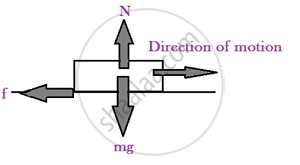From free body diagram, N = mg
Therefore, F_a = f= μN = μmg = 0.25 × 30 × 9.8 = 73.5 N

Problem 2: A homogenous chain of length L lies on a table. What is the maximum length l of the part of the chain hanging over the table if the coefficient of friction between the chain and the table is u, the chain remaining at rest with the table?Solution.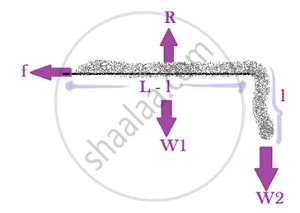Let ρ be the mass per unit length of the chain
Ρ = m/l or, m = ρl
Weight of hanging part = W2 = ρlg
Weight of chain over the table = W1 = ρ(L-l)g

For equilibrium,
R = ρ(L-l)g --------------- (i)
f= W2 = ρlg
μR = ρlg -------------------(ii)

(i) Divided by (ii) gives
1/μ = (L-l)/l
l = μL – μl
l + μl = μL
Therefore, l = (μL)/( μ + 1)

Friction- A boon or Bane against motion
Friction is a boon because of its advantages like:

• Friction helps in walking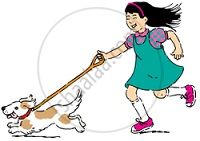When we walk, we push the ground backwards with one foot. According to Newton’s Third law, there is an equal and opposite force exerted by the ground. This force is exerted on a comparatively smaller mass i.e. our foot. So, acceleration is increases. Hence the other foot gets accelerated.
If there is no friction, we will slip and can’t walk.

• Friction helps in movement of automobiles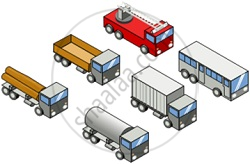Friction is a bane because of its disadvantages:

• A good amount of useful energy is wasted as heat in various machine parts

• Noise produced in machines

• Engines of automobiles consume more fuel

Methods to reduce Friction:

• Use of Lubricants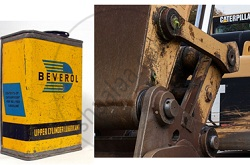Use of Grease:-

• Use of Ball bearing

• Ball bearings are kind of rolling elements that use small freely rotating metal balls which reduce friction.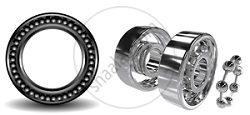Design modification of different parts of machine to reduce friction.

If you would like to contribute notes or other learning material, please submit them using the button below.